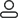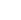Language
0Login|Sign up
Blog >> Blog Details Page

# Characteristic impedance of PCB in high speed design

Posted:04:45 PM February 19, 2019 writer: G

The key to making a board a "controllable impedance board" is to make the characteristic impedance of all lines meet a specified value, typically between 25 ohms and 70 ohms. In a multilayer circuit board, the key to good transmission line performance is to keep its characteristic impedance constant throughout the line.

But what exactly is the characteristic impedance? The easiest way to understand the characteristic impedance is to see what the signal is experiencing during transmission. This is similar to the microwave transmission shown in Figure 1 when moving along a transmission line having the same cross section. Suppose that a 1 volt voltage step wave is applied to the transmission line, such as connecting a 1 volt battery to the front end of the transmission line (which is located between the transmission line and the loop). Once connected, the voltage wave signal follows the line at the speed of light. Propagation, its speed is usually about 6 inches / nanosecond. Of course, this signal is indeed the voltage difference between the transmit line and the loop, which can be measured from any point on the transmit line and the point at which the loop meets. Figure 2 is a schematic diagram of the transmission of the voltage signal.

Zen's approach is to "generate a signal" and then propagate along the transmission line at 6 inches per nanosecond. The first 0.01 nanosecond advances by 0.06 inches, when the transmission line has excess positive charge and the loop has excess negative charge. It is these two charge differences that maintain a voltage difference of 1 volt between the two conductors. The two conductors in turn constitute a capacitor.

In the next 0.01 nanoseconds, the voltage of a 0.06 inch transmission line is adjusted from 0 to 1 volt. This must add some positive charge to the transmission line and add some negative charge to the receiving line. For every 0.06 inch of movement, more positive charge must be applied to the transmission line and more negative charge added to the loop. Every 0.01 nanoseconds, another segment of the transmission line must be charged, and then the signal begins to propagate along this segment. The charge is from the battery at the front end of the transmission line, and as it moves along this line, the continuous portion of the transmission line is charged, thus creating a voltage difference of 1 volt between the transmission line and the loop. Every 0.01 nanoseconds advance, some charge (±Q) is obtained from the battery, and a constant current (±Q) flowing out of the battery at a constant time interval (±t) is a constant current. The negative current flowing into the loop is actually equal to the positive current flowing out, and just at the front end of the signal wave, the alternating current passes through the capacitor composed of the upper and lower lines, ending the entire cycle.

Line impedance

For batteries, the signal propagates along the transmission line and charges a continuous 0.06 inch transmission line segment every 0.01 nanoseconds. When a constant current is obtained from the power supply, the transmission line looks like a resistor and its impedance value is constant, which can be called the "surge" impedance of the transmission line.

Similarly, when the signal propagates along the line, which current can increase the voltage of this step to 1 volt within 0.01 nanoseconds before the next step? This involves the concept of instantaneous impedance.

From the perspective of the battery, if the signal propagates along the transmission line at a steady speed and the transmission lines have the same cross section, then the same amount of charge is required further in advance in 0.01 nanoseconds to produce the same signal voltage. When traveling along this line, the same instantaneous impedance is produced, which is considered a characteristic of the transmission line and is called the characteristic impedance. If the signal has the same characteristic impedance at each step of the transfer process, then the transmission line can be considered a controllable impedance transmission line.

Instantaneous impedance or characteristic impedance is very important for signal transmission quality. During the transfer process, if the impedance of the next step is equal to the impedance of the previous step, the work can proceed smoothly, but if the impedance changes, some problems will occur.

In order to achieve the best signal quality, the internal connection is designed to maintain impedance stability during signal transmission. First, the characteristic impedance of the transmission line must be kept stable. Therefore, the production of controllable impedance boards becomes more and more important. In addition, other methods such as minimizing the length of the remaining line, removing the end, and using the entire line are also used to maintain the stability of the transient impedance in signal transmission.

Calculation of characteristic impedance

A simple characteristic impedance model: Z = V / I, Z represents the impedance of each step in the signal transmission process, V represents the voltage when the signal enters the transmission line, and I represents the current. I = ± Q / ± t, Q represents the amount of electricity, and t represents the time of each step.

Power (from battery): ± Q = ± C × V, C for capacitance, V for voltage. The capacitance can be derived using the transmission line unit length capacity CL and the signal transmission speed v. The length value of the unit pin is taken as the speed, and multiplied by the time t required for each step, the formula is obtained: ±C=CL×v×(±)t. Combining the above, we can get the characteristic impedance: Z= V/I=V/(±Q/±t)=V/(±C×V/±t)=V/(CL×v×(±)t×V/±t)=1/(CL×v )

It can be seen that the characteristic impedance is related to the transmission line unit length capacity and signal transmission speed. In order to distinguish the characteristic impedance from the actual impedance Z, we add 0 after Z. The characteristic impedance of the transmission line is: Z0 = 1 / (CL × v).

If the transmission line unit length capacity and signal transmission speed remain the same, then the transmission line characteristic impedance remains unchanged. This simple description links the common sense of capacitance to the newly discovered characteristic impedance theory. If the transmission line unit length capacity is increased, such as a thickened transmission line, the transmission line characteristic impedance can be reduced.

Characteristic impedance measurement

When the battery is connected to the transmission line (if the impedance is 50 ohms at the time), connect the ohmmeter to the 3 foot long RG58 cable. How do you measure the infinite impedance? The impedance of any transmission line is related to time. If you measure the impedance of the cable in a shorter time than the cable reflection, you measure the "surge" impedance, or characteristic impedance. However, if you wait for a long enough time until the energy is reflected back and received, the impedance can be found to change. In general, the impedance value will reach a stable limit after rebounding up and down.

For a 3 foot cable, the impedance measurement must be completed within 3 nanoseconds. This can be done with the TDR (Time Domain Reflectometer), which measures the dynamic impedance of the transmission line. If you measure the impedance of a 3 foot cable in 1 second, the signal will reflect back and forth millions of times, so you get a different "surge" impedance.

809 1 0 1
• PCB
Prototype
• PCB
Assembly
• SMD
Stencil
 Dimensions: (mm) × Quantity: (pcs) 5 5 10 15 20 25 30 40 50 75 100 120 150 200 250 300 350 400 450 500 600 700 800 900 1000 1500 2000 2500 3000 3500 4000 4500 5000 5500 6000 6500 7000 7500 8000 9000 10000 Other Quantities:(quantity*length*width is greater than 10㎡) OK Layers: 1 2 4 6 8 10 12 Thickness: 0.6 mm 0.8 mm 1.0 mm 1.2 mm 1.6 mm 2.0 mm 2.5 mmQuote now
###### Recommended Article:
[P-E15079AXA44] Ee of the oe of the oe of the o
Copy of the cause of the oe of the oe of the order the order Copy of the...

11:34 AM August 31, 2020